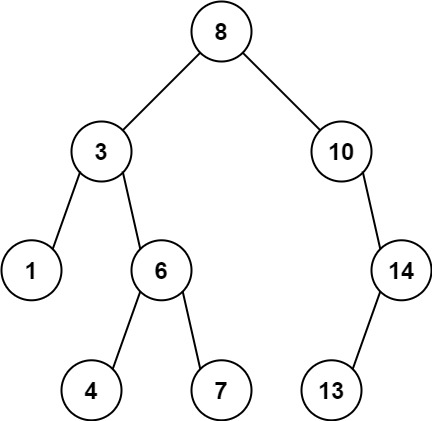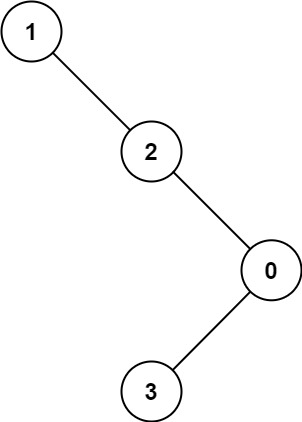## C# || How To Find Maximum Difference Between Node and Ancestor In Binary Tree Using C#The following is a module with functions which demonstrates how to find the maximum difference between node and ancestor in binary tree using C#.

1. Max Ancestor Diff – Problem Statement

Given the root of a binary tree, find the maximum value v for which there exist different nodes a and b where v = |a.val – b.val| and a is an ancestor of b.

A node a is an ancestor of b if either: any child of a is equal to b or any child of a is an ancestor of b.

Example 1:``` Input: root = [8,3,10,1,6,null,14,null,null,4,7,13] Output: 7 Explanation: We have various ancestor-node differences, some of which are given below : |8 - 3| = 5 |3 - 7| = 4 |8 - 1| = 7 |10 - 13| = 3 Among all possible differences, the maximum value of 7 is obtained by |8 - 1| = 7. ```

Example 2:``` Input: root = [1,null,2,null,0,3] Output: 3 ```

2. Max Ancestor Diff – Solution

The following is a solution which demonstrates how to find the maximum difference between node and ancestor in binary tree.

``` 2. Max Ancestor Diff - Solution C# // ============================================================================ // Author: Kenneth Perkins // Date: Feb 14, 2023 // Taken From: http://programmingnotes.org/ // File: Solution.cs // Description: Demonstrates how to find maximum difference node and ancestor // ============================================================================ /** * Definition for a binary tree node. * public class TreeNode { * public int val; * public TreeNode left; * public TreeNode right; * public TreeNode(int val=0, TreeNode left=null, TreeNode right=null) { * this.val = val; * this.left = left; * this.right = right; * } * } */ public class Solution { public int MaxAncestorDiff(TreeNode root) { if (root == null) { return 0; } return Traverse(root, root.val, root.val); } public int Traverse(TreeNode node, int curMax, int curMin) { // if encounter leaves, return the max-min along the path if (node == null) { return curMax - curMin; } // else, update max and min // and return the max of left and right subtrees curMax = Math.Max(curMax, node.val); curMin = Math.Min(curMin, node.val); int left = Traverse(node.left, curMax, curMin); int right = Traverse(node.right, curMax, curMin); return Math.Max(left, right); } }// http://programmingnotes.org/ 123456789101112131415161718192021222324252627282930313233343536373839404142 // ============================================================================//    Author: Kenneth Perkins//    Date:   Feb 14, 2023//    Taken From: http://programmingnotes.org///    File:  Solution.cs//    Description: Demonstrates how to find maximum difference node and ancestor// ============================================================================/** * Definition for a binary tree node. * public class TreeNode { *     public int val; *     public TreeNode left; *     public TreeNode right; *     public TreeNode(int val=0, TreeNode left=null, TreeNode right=null) { *         this.val = val; *         this.left = left; *         this.right = right; *     } * } */public class Solution {    public int MaxAncestorDiff(TreeNode root) {        if (root == null) {            return 0;        }        return Traverse(root, root.val, root.val);    }     public int Traverse(TreeNode node, int curMax, int curMin) {        // if encounter leaves, return the max-min along the path        if (node == null) {            return curMax - curMin;        }        // else, update max and min        // and return the max of left and right subtrees        curMax = Math.Max(curMax, node.val);        curMin = Math.Min(curMin, node.val);        int left = Traverse(node.left, curMax, curMin);        int right = Traverse(node.right, curMax, curMin);        return Math.Max(left, right);    }}// http://programmingnotes.org/ ```

QUICK NOTES:
The highlighted lines are sections of interest to look out for.

The code is heavily commented, so no further insight is necessary. If you have any questions, feel free to leave a comment below.

Once compiled, you should get this as your output for the example cases:

``` 7 3 ```